# Class 9 Maths Chapter 10 Circles MCQs

Class 9 Maths Chapter 10 Circles MCQs are available online, here with answers. These are the objective questions prepared, as per the CBSE syllabus and NCERT curriculum. The multiple-choice questions are given here chapter-wise, with detailed explanations. Also, check Important Questions for Class 9 Maths.

## MCQs on Class 9 Maths Chapter 10 Circles

Solve the MCQs on circles given here with four multiple options and choose the right answer.

1) The center of the circle lies in______ of the circle.

a. Interior

b. Exterior

c. Circumference

d. None of the above

2) The longest chord of the circle is:

b. Arc

c. Diameter

d. Segment

3) Equal _____ of the congruent circles subtend equal angles at the centers.

a. Segments

c. Arcs

d. Chords

Explanation: See the figure below: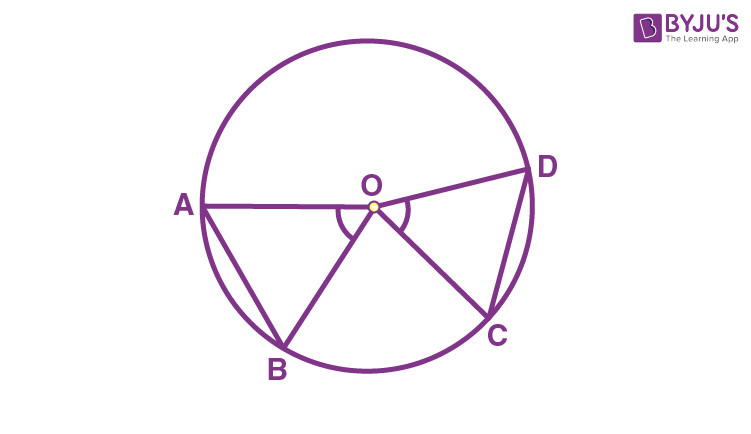Let ΔAOB and ΔCOD are two triangles inside the circle.

OA = OC and OB = OD (radii of the circle)

AB = CD (Given)

So, ΔAOB ≅ ΔCOD (SSS congruency)

∴ By CPCT rule, ∠AOB = ∠COD.

Hence, this prove the statement.

4) If chords AB and CD of congruent circles subtend equal angles at their centres, then:

a. AB = CD

b. AB > CD

d . None of the above

Explanation: Take the reference of the figure from above question.

In triangles AOB and COD,

∠AOB = ∠COD (given)

OA = OC and OB = OD (radii of the circle)

So, ΔAOB ≅ ΔCOD. (SAS congruency)

∴ AB = CD (By CPCT)

5) If there are two separate circles drawn apart from each other, then the maximum number of common points they have:

a. 0

b. 1

c. 2

d. 3

6) The angle subtended by the diameter of a semi-circle is:

a. 90

b. 45

c. 180

d. 60

Explanation: The semicircle is half of the circle, hence the diameter of the semicircle will be a straight line subtending 180 degrees.

7) If AB and CD are two chords of a circle intersecting at point E, as per the given figure. Then: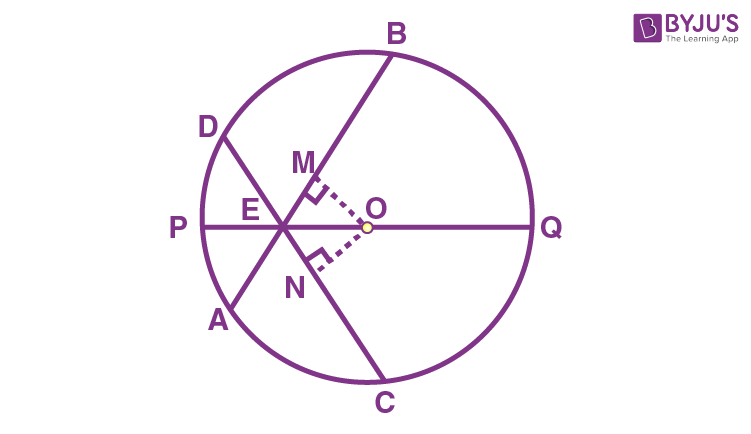a.∠BEQ > ∠CEQ

b. ∠BEQ = ∠CEQ

c. ∠BEQ < ∠CEQ

d. None of the above

Explanation:

OM = ON (Equal chords are always equidistant from the centre)

OE = OE (Common)

∠OME = ∠ONE (perpendiculars)

So, ΔOEM ≅ ΔOEN (by RHS similarity criterion)

Hence, ∠MEO = ∠NEO (by CPCT rule)

∴ ∠BEQ = ∠CEQ

8) If a line intersects two concentric circles with centre O at A, B, C and D, then:

a. AB = CD

b. AB > CD

c. AB < CD

d. None of the above

Explanation: See the figure below: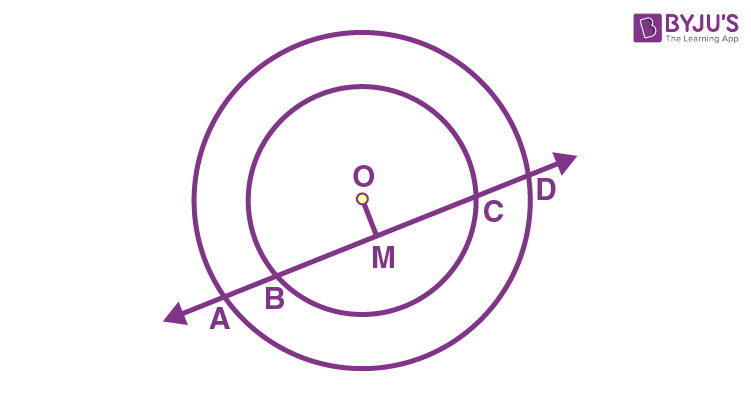From the above fig., OM ⊥ AD.

Therefore, AM = MD — 1

Also, since OM ⊥ BC, OM bisects BC.

Therefore, BM = MC — 2

From equation 1 and equation 2.

AM – BM = MD – MC

∴ AB = CD

9) In the below figure, the value of ∠ADC is: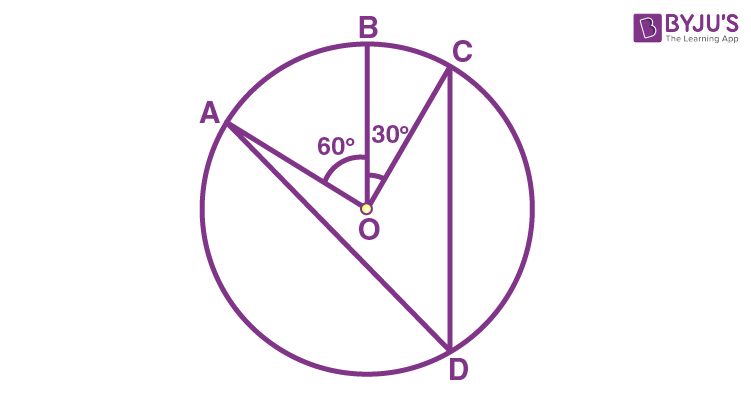a. 60°

b. 30°

c. 45°

d. 55°

Explanation: ∠AOC = ∠AOB + ∠BOC

So, ∠AOC = 60° + 30°

∴ ∠AOC = 90°

An angle subtended by an arc at the centre of the circle is twice the angle subtended by that arc at any point on the rest part of the circle.

So,

= 1/2 × 90° = 45°

10) In the given figure, find angle OPR.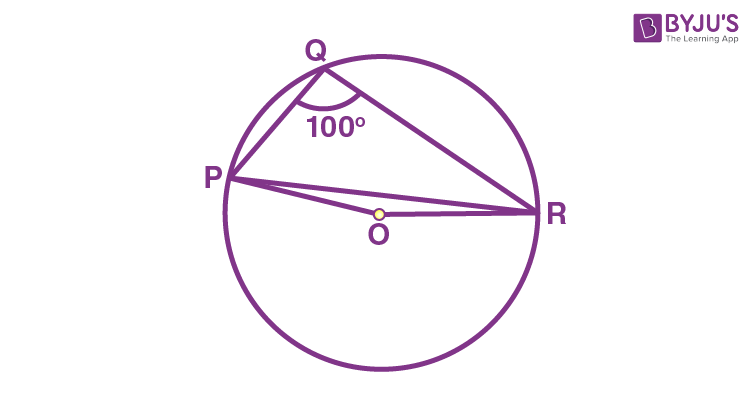a. 20°

b. 15°

c. 12°

d. 10°

Explanation: The angle subtended by major arc PR at the centre of the circle is twice the angle subtended by that arc at point, Q, on the circle.

So, ∠POR = 2 × ∠PQR, here ∠POR is the exterior angle

We know the values of angle PQR as 100°

So, ∠POR = 2 × 100° = 200°

∴ ∠ROP = 360° – 200° = 160°   [Full rotation: 360°]

Now, in ΔOPR,

OP and OR are the radii of the circle

So, OP = OR

Also, ∠OPR = ∠ORP

By angle sum property of triangle, we know:

∠ROP + ∠OPR + ∠ORP = 180°

∠OPR + ∠OPR = 180° – 160°

As, ∠OPR = ∠ORP

2∠OPR = 20°

Thus, ∠OPR = 10°

11) In the given figure, ∠AOB = 90º and ∠ABC = 30º, then ∠CAO is equal to: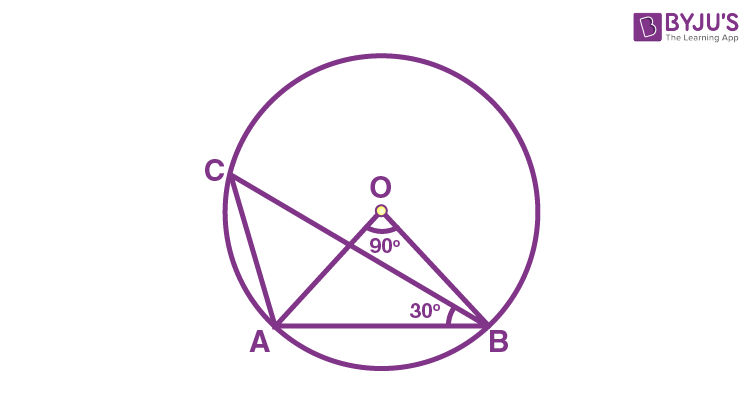(a) 30º

(b) 45º

(c) 60º

(d) 90º

Explanation:

Given that ∠AOB = 90º and ∠ABC = 30º

OA = OB (Radii of the circle)

Let x= ∠OAB = ∠OBA = x

In the triangle OAB,

∠OAB + ∠OBA + ∠AOB = 180° (By using the angle sum property of triangle)

⇒ x + x + 90° = 180°

⇒ 2x = 180° – 90°

⇒ x =  90°/ 2 = 45°

Therefore, ∠OAB = 45° and ∠OBA = 45°

By using the theorem, “ the angles subtended by arcs at the centre of the circle double the angle subtended at the remaining part of the circle”, we can write

∠AOB = 2∠ACB

This can also be written as,

∠ACB =  ½  ∠AOB =  (½) × 90° = 45°

Now, apply the angle sum property of triangle on the triangle ABC,

∠ACB + ∠BAC + ∠CBA = 180°

∠ACB + [∠BAO + ∠CAO] + ∠CBA = 180° (As,∠BAC = ∠BAO + ∠CAO)

Now, substitute the known values, we get

45° + (45° + ∠CAO) + 30° = 180°

∠CAO = 180°- (30° + 45° + 45°)

∠CAO = 180°-120°

∠CAO = 60°

Hence, ∠CAO is equal to 60°.

12) ABCD is a cyclic quadrilateral such that AB is a diameter of the circle circumscribing it and ∠ADC = 140º, then ∠BAC is equal to:

(a) 30º

(b) 40º

(c) 50º

(d) 80º

Explanation: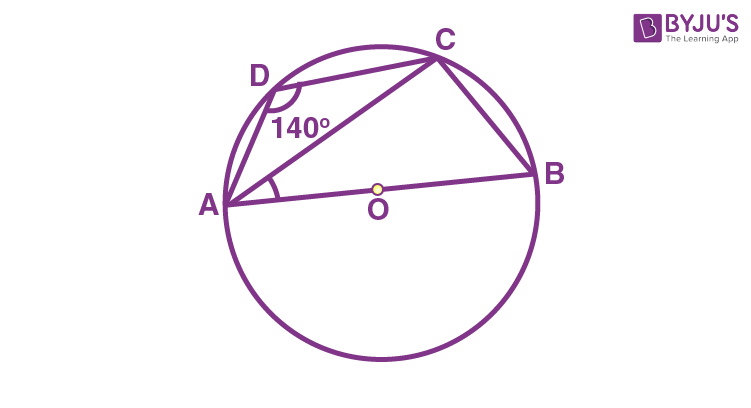We know that the sum of opposite angles of a cyclic quadrilateral is 180°.

Hence, ∠ADC + ∠ABC = 180°

Now, substitute ∠ADC = 140º in the above equation, we get

140° + ∠ABC = 180°

∠ABC = 180° – 140° = 40°

since the angle subtended by a diameter at the circumference of the circle, is 90°

Hence,  ∠ACB = 90°

By using the angle property of triangle in the triangle, ABC,

∠CAB + ∠ABC + ∠ACB = 180°

∠CAB + 40° + 90° = 180°

∠CAB = 180° – 90° – 40°

∠CAB = 50°

Therefore, ∠CAB or ∠BAC =50°.

13) In the given figure, if ∠OAB = 40º, then ∠ACB is equal to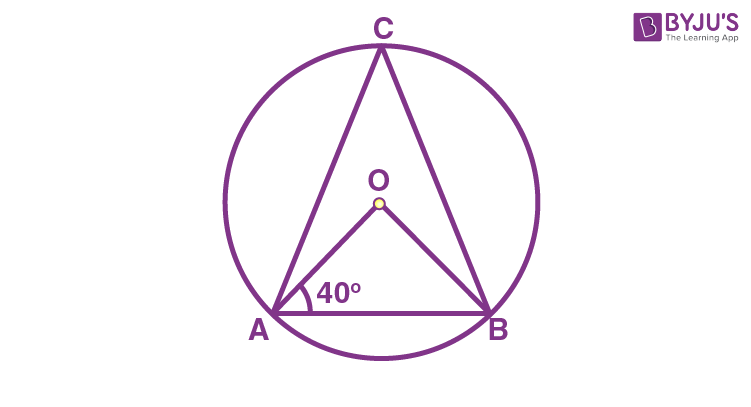(a) 40º

(b) 50º

(c) 60º

(d) 70º

Explanation:

Given that ∠OAB = 40º,

In  the triangle OAB,

Since, the angles opposite to equal sides are equal,

∠OAB = ∠OBA (i.e.) ∠OBA = 40°

Now, by using the angle sum property of triangle, we can write

∠AOB + ∠OBA + ∠BAO = 180°

Now, substitute the known values,

∠AOB + 40° + 40° = 180°

∠AOB = 180 – 80° = 100°

∠AOB = 2 ∠ACB  (Since, the angle subtended by an arc at the centre is twice the angle subtended by it at the remaining part of the circle)

∠ACB = ½ ∠AOB

Hence, ∠ACB = 100°/2 = 50°.

14) In the given figure, if ∠ABC = 20º, then ∠AOC is equal to: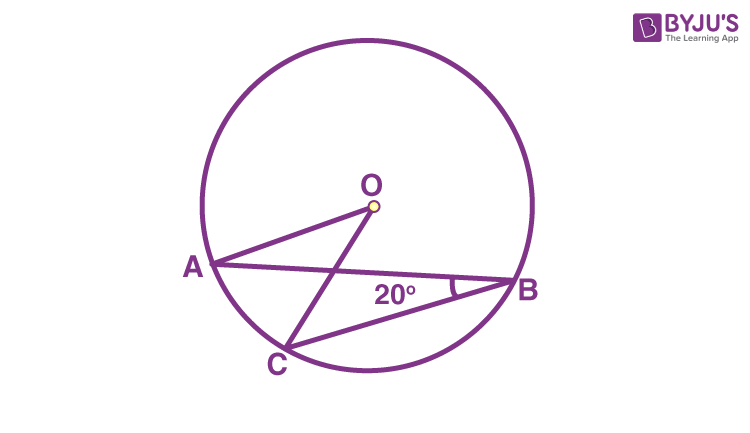(a) 10º

(b) 20º

(c) 40º

(d) 60º

Explanation:

Given that, ∠ABC = 20º.

∠AOC = 2∠ABC (since the angle subtended by an arc at the centre of the circle is double the angle subtended at the remaining part.)

Now, substitute the values, we get

∠AOC = 2 × 20°

Therefore, ∠AOC = 40°.

15) In the given figure, if OA = 5 cm, AB = 8 cm and OD is perpendicular to AB, then CD is equal to: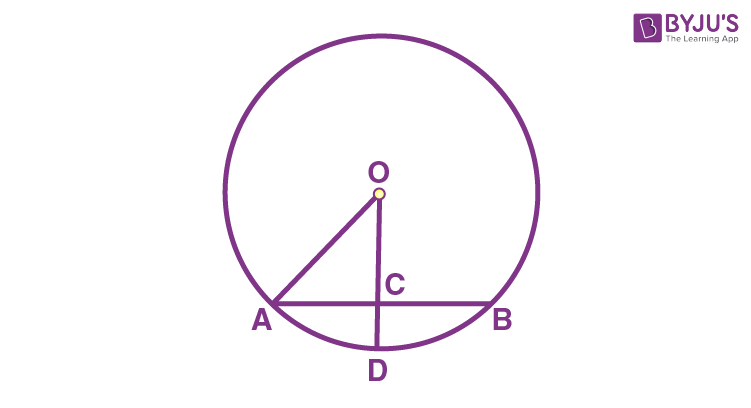(a) 2 cm

(b) 3 cm

(c) 4 cm

(d) 5 cm

Explanation:

From the given diagram, we can observe that OC is perpendicular to chord AB. Therefore, OC bisects the chord AB Hence. AC=CB

Also,

AC+CB=AB

AC+CB=8

2AC = 8 (Since, AC = CB)

AC = 8/2 = 4 cm

As, the triangle OCA is a right-angled triangle, by using Pythagoras theorem, we can write

AO2=AC2+OC2

52=42+OC2

52−42=OC2

OC2=9

OC=3 cm

As, OD is the radius of the circle, OA=OD=5cm

CD=OD-OC

CD = 5-3 = 2 cm

Hence, the value of CD is equal to 2cm.

16) In the given figure, BC is the diameter of the circle and ∠BAO = 60º. Then ∠ADC is equal to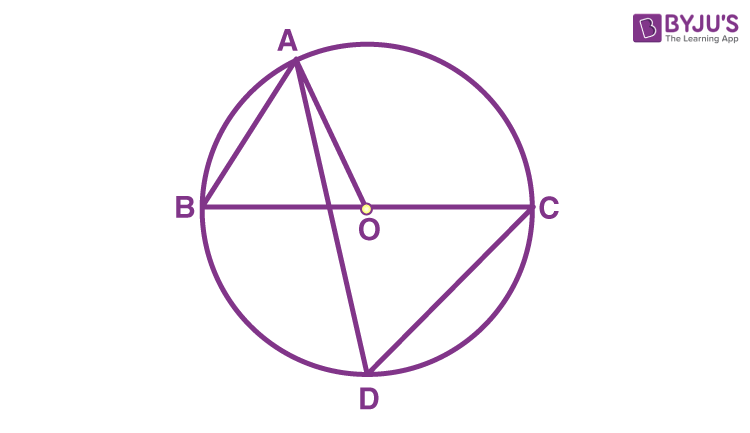(a) 30º

(b) 45º

(c) 60º

(d) 120º

Explanation:

Given that ∠BAO = 60°

Since OA = OB,∠OBA = 60°

Then ∠ADC = 60° (As, the angles in the same segment are equal).

17) In the given figure, if ∠DAB = 60º, ∠ABD = 50º, then ∠ACB is equal to: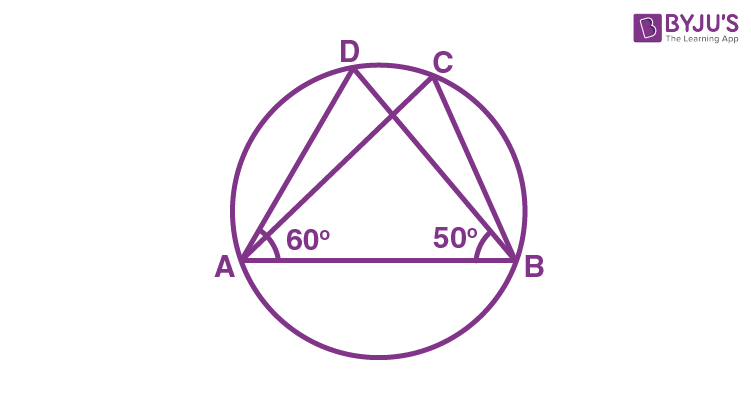(a) 50º

(b) 60º

(c) 70º

(d) 80º

Explanation:

Given that, ∠DAB = 60º, ∠ABD = 50º

By using the angle sum property in the triangle ABD

∠ADB=∠ACB (Since the angles subtended at the circumference by the same arc are equal)

We know that the angles subtended at the circumference by the same arc are equal.

∠ACB =70º

18) In the given figure, if AOB is a diameter of the circle and AC = BC, then ∠CAB is equal to: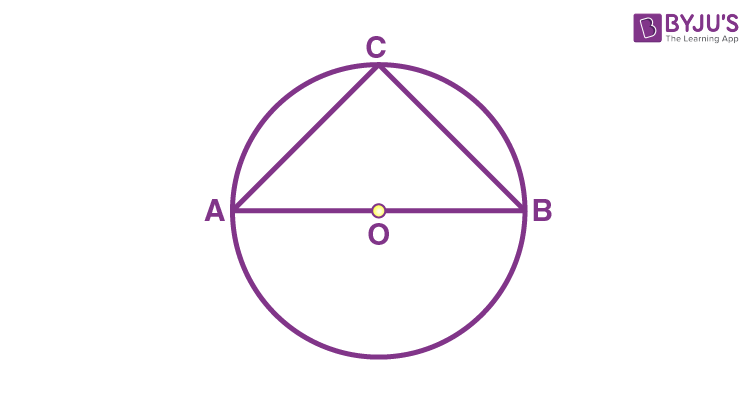(a) 30º

(b) 45º

(c) 60º

(d) 90º

Explanation:

We know that the angle at circumference subtended by the diameter of the circle is the right angle.

Hence, ∠ACB = 90°

Also, given that AC = BC

Therefore, ∠CAB = ∠CBA (As, the angles opposite to equal sides are also equal)

Now, by using the angle sum property of triangle in ∆ACB, we can write

∠CAB + ∠ABC + ∠BCA = 180°

∠CAB + ∠CAB + 90° = 180°

2∠CAB = 180° – 90°

∠CAB = 45°

Therefore, ∠CAB is equal to 45°.

19) If AB = 12 cm, BC = 16 cm and AB is perpendicular to BC, then the radius of the circle passing through the points A, B and C is:

(a) 6 cm

(b) 8 cm

(c) 10 cm

(d) 12 cm

Explanation:

Given that AB = 12 cm, BC = 16 cm and AB is perpendicular to BC.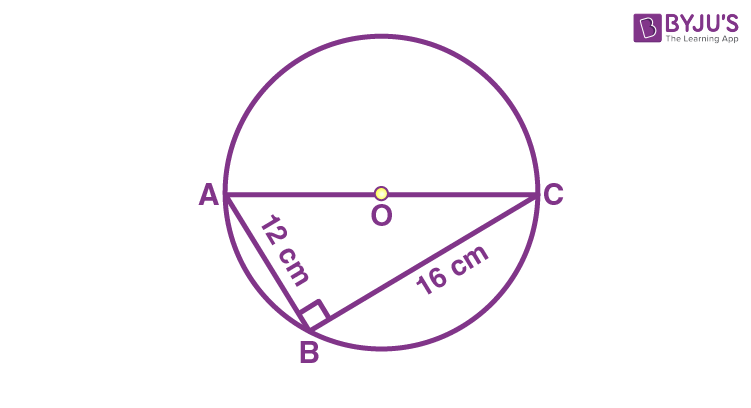Hence, AC is the diameter of the circle passing through points A, B and C.

Hence, ABC is a right-angled triangle.

Thus by using the Pythagoras theorem:

AC2 = (CB)2 + (AB)2

⇒ AC2 = (16)2 + (12)2

⇒ AC2 = 256 + 144

⇒ AC2 = 400

Hence, the diameter of the circle, AC = 20 cm.

Thus, the radius of the circle is 10 cm.

20) AD is the diameter of a circle and AB is a chord. If AD = 34 cm, AB = 30 cm, the distance of AB from the centre of the circle is

(a) 4 cm

(b) 8 cm

(c) 15 cm

(d) 17 cm

Explanation:

Given that, Diameter, AD = 34 cm.

Chord, AB = 30 cm.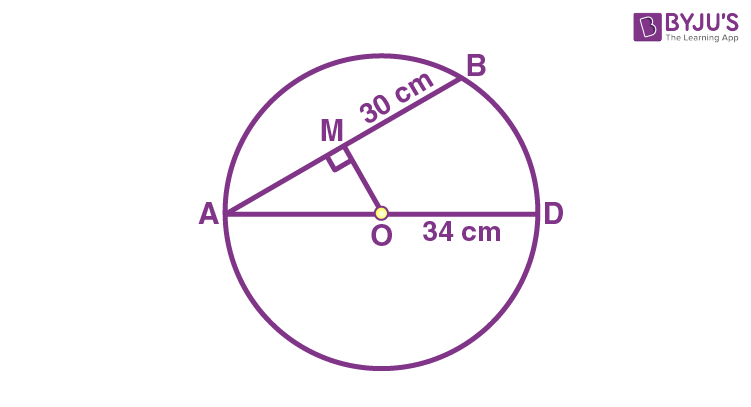Hence, the radius of the circle, OA = 17 cm

Now, consider the figure.

From the centre “O”. OM is perpendicular to the chord AB.

(i.e) OM ⊥ AM

AM =  ½  AB

AM =  ½ (30) = 15 cm

Now by using the Pythagoras theorem in the right triangle AOM,

AO2 = OM2 + AM2

OM2 = AO2– AM2

OM2= 172 – 152

OM2 = 64

OM = √64

OM = 8 cm

Stay tuned with BYJU’S – The Learning App and download the app today to get more class-wise concepts.

1. Jaikrish Vetriselvi

This is awesome where I come to know the correct answer and too with explanation but in the last sum after fine the value of ANGLE POR as 200. The why did you subtracted with 360 l don’t know can you kindly clarify my doubt please

1. lavanya

∠POR is the angle formed by major arc PR with center o.
Therefore, it is equal to twice of ∠PQR
Now, ∠POR or ∠ROP with minor arc PR, will be:
∠ROP = 360° – 200° = 160° ; 360° is the full rotation at center o.

2. Aaryan Gupta

we canceled 360 degree as it is forming a complete angle and we and we have to find the angle OPR. First we used complete angle theorem and then angle sum property

2. Yash bhardwaj

Good well questions…..they will guide me very well thank you byjus…..I am download byjus app today….. I am study in this app this app is very brilliant plus making efficiency for stuyding……….thanks a lot byjus you will help me in covid _ 19 in stuyding…..

3. Great content!!!

4. This is great that the question is in mcq form with answers and explanation as well 😘😘

5. Ratnali Boritkar

Nice questions & explanation as well.